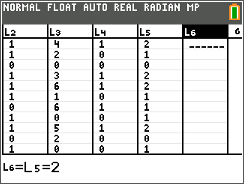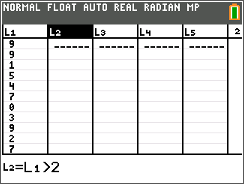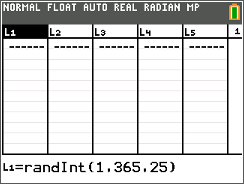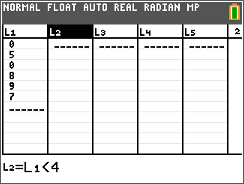# Activities

••• ##### Subject Area

• Math: Statistics: Probability and Random Variables

• ##### Author9-12

45 Minutes

• ##### Device
• TI-84 Plus
• TI-84 Plus Silver Edition
•TI-84 Plus C Silver Edition
•TI-84 Plus CE
• ##### Report an Issue

In It to Win It!#### Activity Overview

Students will calculate the experimental probability of winning a basketball game by shooting two free-throws using a random integer simulation.

#### Key Steps

•A 70% free throw shooter is at the foul line with no time left on the clock. What is the probability that she will make both free throws to win the game?

This problem is simulated using lists of random integers. The randInt( command is used to generate the free throws and the Boolean test commands < and > are used to determine if the free throw is missed or completed. Students will compare their experimental probability with the other experimental probabilities in the class and also with the theoretical probability.

•For the second problem, students will investigate the famous problem – If there are 25 unrelated people in a room, what is the probability that two of them will share a birthday?

They will use the random number generator and the SortA command to simulate this scenario. After doing 10 simulations, students will the number of times the room shared a birthday by divide 10 to calculate the experimental probability. They will compare this to others in the classroom and the theoretical probability.

•In the extension, students will use what they’ve learned from Problems 1 and 2 to calculate the experimental probability of a batter walking to first base, given the percentage of strikes that the pitcher throws. They will need to use the randInt command and keep a running tally of whether the batter walked for each trial.